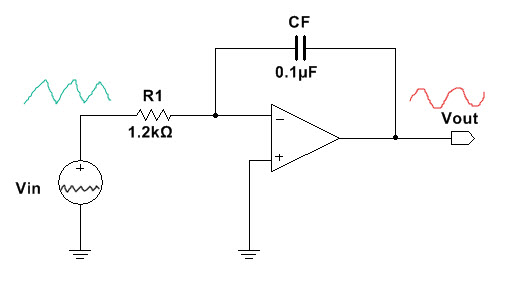# How to design Basic or Ideal Op-Amp Integrator Circuit

An integrator circuit is a circuit that outputs voltage that is integration of the input voltage. An integrator circuit can be passive or active. A passive integrator circuit is build using passive components like resistor and capacitor. An active integrator circuit is build using op-amps or transistors. Here ideal or basic active integrator design using op-amp is illustrated. The behavior of integrator circuit to different types of inputs such as square wave, triangle wave and sine wave is illustrated. Bias compensation resistor is added to the basic op-amp integrator is shown. Frequency response of the ideal or basic op-amp circuit is shown. Finally the limitation of a basic or ideal active op-amp based integrator is explained.

Ideal Op-Amp Integrator

An ideal op-amp integrator circuit or basic op-amp integrator circuit diagram is shown below. The capacitor C1 is connected from the output back to input.

We can derive the following equation for the output voltage Vout,

Thus for the integrator circuit, the output voltage is -1/R1CF times the integral of the input voltage. The negative sign indicates that the output voltage is shifted 180 degree out of phase relative to the input voltage.

The value R1CF is called the time constant of the integrator. For example, if R1=2.4KOhm and CF=0.1uF then time constant is R1*CF = 2.4KOhm*0.1uF=0.1ms.

Also in the above equation t is the time period which is the inverse of the frequency of the input signal. If for example the input signal frequency is 1KHz then the time period t of integration is 1ms.

Application of Integrator circuit

Some of the application of integrator circuit includes solving differential equation, are used in analog computers, are used in analog to digital converters, for signal wave shaping circuits, function generator or waveform generator, in frequency modulation circuits etc.

Square Wave to Triangle Wave

It can be mathematically proved that if the input signal to the integrator circuit is a square wave then the output from the integrator circuit will be a triangle wave. The circuit shown below has a square input and triangle wave output.

The input and output signal waveform is shown below.

Triangle Wave to Sine Wave

If the input to the integrator circuit is a triangle wave then the output will be a sine wave.The input and output signal waveform is shown below.

Sine Wave to Cosine Wave

If the input signal to integrator circuit is a sine wave then we get a 90 degree shifted cosine wave.

The waveform of the input and output signal for this case is shown below.

Ideal Integrator with Bias Compensation

In most integrator circuit a bias compensation resistor Rb is connected to the non-inverting terminal. The value of Rb is equal to the resistor R1. The following circuit diagram shows op-amp integrator circuit with bias compensation.

We will the same output as before with bias compensation as in the above cases. The bias compensation resistor is just there to correct any imbalance in the inputs of the integrator circuit.

Frequency Response of Ideal Integrator

By taking the Laplace transform of the input and output voltage equation of the integrator shown above we can derive the magnitude of the gain as follows,

$$A= \frac{1}{2 \pi R_{1}C_{F}}$$

We can plot the frequency response of ideal integrator in circuit simulation software like multisim. The following shows the magnitude(gain in dB) vs frequency and the phase response of the ideal integrator circuit.

The magnitude response graph is re-shown below.

We can see that the at very low frequencies the gain is very high. The gain is very high at low frequencies because at low frequency the capacitive reactance is high. In this situation, the circuit behaves open circuit and the gain of open circuit or open loop op-amp circuit is very high(infinite).

If fb is the breakover frequency at which the gain of the op-amp becomes unity, that is 0dB then we can derive the frequency fb as being,

$$f_{b} = \frac{1}{2 \pi R_{1}C_{F}}$$

Limitation and Errors in basic or ideal Op-Amp Integrator

Like any other op-amp circuits, in absence of any input signal, there exists some input offset voltage and input bias current at the input terminal of op-amp integrator circuit. The gain of op-amp is high and therefore the input offset voltage gets amplified and this results in voltage error at the output. Similarly, the input bias current also adds up error in the output voltage because the current charges the capacitor which effects the output voltage. The net effect of these two input bias components is that the output ramps up and down which depends on the polarities of the offset voltage and the bias current. Furthermore, sometimes due to the error components the op-amp may be driven into saturation and it will be difficult to pull out the op-amp output voltage level out of saturation.

Another limitation of basic or ideal op-amp integrator circuit is that the bandwidth is very small. So we can only apply input signals within small frequency range.

The solution to basic or ideal op-amp integrator is to use a practical integrator circuit which is explained in How to design practical Op-Amp Integrator Circuit. For actual design see How to Design LM358 Op-Amp Practical Integrator.